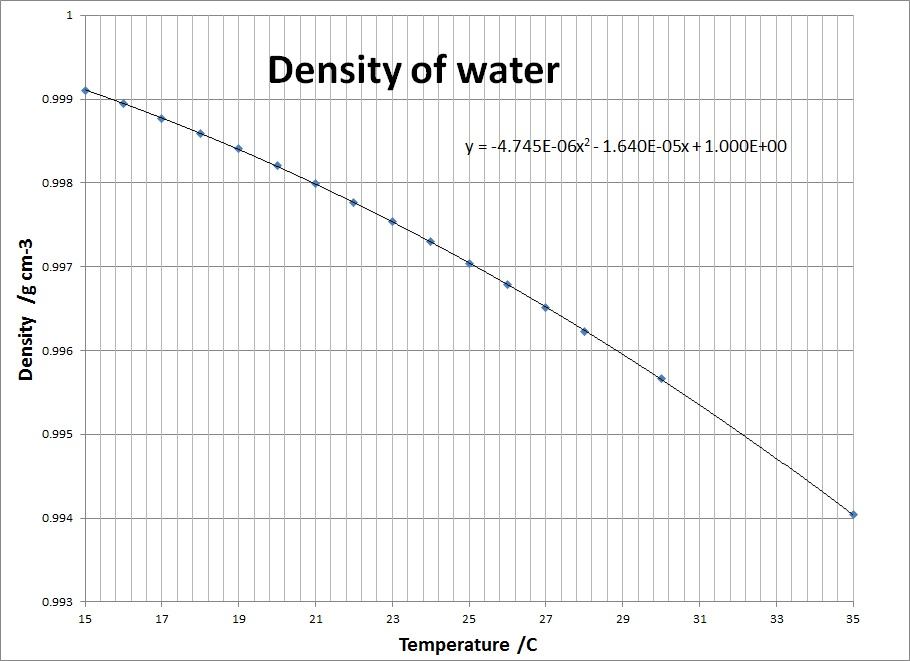Experiment 2: Calibration of a pipette

Introduction
An ordinary laboratory pipette may be expected to deliver its nominal volume with good precision and good accuracy if it is used in the way recommended. In this experiment we investigate the precision and accuracy of such a pipette by making accurate determinations of the mass of water it delivers in repeated operations.

Materials
10.0-cm3 pipette, 50-cm3 beaker, 250-cm3 Erlenmeyer flask, thermometer, pipette filler, graph paper.

1. Clean the beaker and the pipette and dry the beaker.
2. Obtained distilled water in the Erlenmeyer flask and let it stand on the bench for about 15 min before determining its temperature.
3. Weigh the beaker on a balance which allows you to determine the mass to the nearest tenth of a milligram (i.e. ± 0.001 g).
4. Fill (use the pipette filler) and discharge the pipette as recommended, into the beaker, and determine the mass of water discharged by taking the difference.
5. Repeat step 4 until you have the results of four (4) such trials.

Note: You do not need to empty and dry the beaker between trials. You should try to spend no more than a half-hour in the balance room so that everyone will have an opportunity to use the balances in the time allotted.

6. Determine the temperature of the water you have pipetted and take the mean of the two temperatures you have measured as the effective temperature of the water during the calibration.
Calculations
1. Find the mean of the four trials. The mean volume can now be determined from the mean mass and the density of water at the temperature you determined in 6 above. However, there is a correction to be made for the upthrust of air on the water during the weighing. In normal day-to-day use of balances we neglect this correction, but now that we are interested in accurate calibration, we will take this into account. The correction occurs because the upthrust of air on the water is greater than the upthrust on the balance weights used in our balances.

This correction is in effect, 1.06 mg for every gram of water weighed, (when it is assumed that the density of air is 0.0012 g cm3 and the density of stainless steel weights is 8.4 g cm3).

2. Correct the mean mass for air buoyancy by adding to the mean mass, 1.06 mg per gram of water discharged by the pipette.

3. Determine the density of water, at the temperature measured in 6 above, from the tables given.4. Find the mean volume of water discharged by the pipette using the formula:

Vo = mass / density

5. Comment on the accuracy of the pipette and show your results to a demonstrator, who will initial the results and make a note of your value.

6. We now want to determine the precision of the pipette, but do not have enough data points to do rigorous statistical analysis. The demonstrator will put you in touch with someone with a pipette with volume fairly close to yours. Copy this person's four data points and perform the statistical analysis to find the standard deviation of the eight data points. You may neglect the correction for air buoyancy in doing the statistical analysis, but you should find a new mean volume for your eight data points.

Table of Mass and Volume of Water obtained in 8 Pipette Trials

 Trial No. Mass of water/g Vol. of water, Vi/cm3 (Vi - Vavg) = di (Vi - Vavg)2 = di2 1 2 3 4 5 6 7 8

The standard deviation, σ, is given by the formula:

σ= √ {∑(di)2 / (N-1)}

7. Write your result for the volume you determined in 4 with the error you have now estimated from the data;
Volume of pipette = Vo (± s)

Comment now, on the accuracy and precision of the pipette.Return to Chemistry, UWI-Mona, Home Page